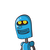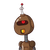# = 182 x 22/7 = 26 x 22 = 572 cm?– A toy is in the form of a cone of radius 3.5 cmmounted on a hemisphere of same radiu

= 182 x 22/7 = 26 x 22 = 572 cm?
– A toy is in the form of a cone of radius 3.5 cm
mounted on a hemisphere of same radius. The
total height of the toy is 15.5 cm. Find the total
surface area of the toy.

$$anyone \: \gamma$$

### 2 thoughts on “= 182 x 22/7 = 26 x 22 = 572 cm?<br />– A toy is in the form of a cone of radius 3.5 cm<br />mounted on a hemisphere of same radiu”

1.$$\Huge\bf\maltese{\underline{\green{Answer°᭄}}}\maltese$$

$$\implies$$$$\large\bf{\underline{\red{VERIFIED✔}}}$$

Total surface area of toy =  Curved surface area of cone +  surface area of hemisphere

Curved  surface area of cone = πrl

=> Where r = 3.5cm,

=> Height = 15.5 − 3.5 = 12cm

And hence, l=12.5cm(by using formula l²=h²+b²)

Therefore C.S.A. of cone = π×3.5×12.5

= 137.5cm²

Surface area of hemisphere =2πr²

=2×π×(3.5)²

=77cm²

Hence T.S.A of toy = 77+137.5

= 214.5cm²

$$\boxed{I \:Hope\: it’s \:Helpful}$$

2.$$\huge\ \maltese{\underline{\red{ANSWER}}}\maltese$$